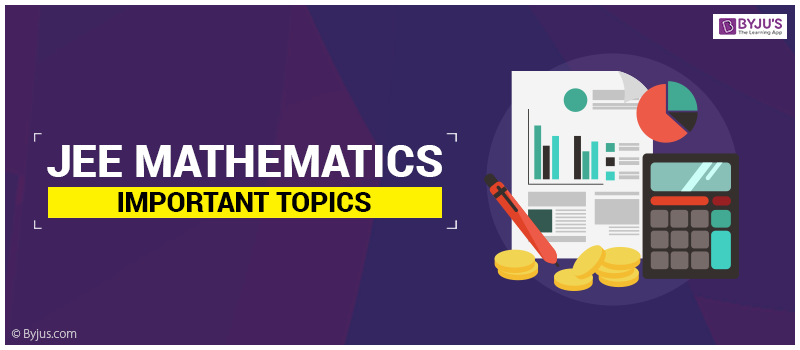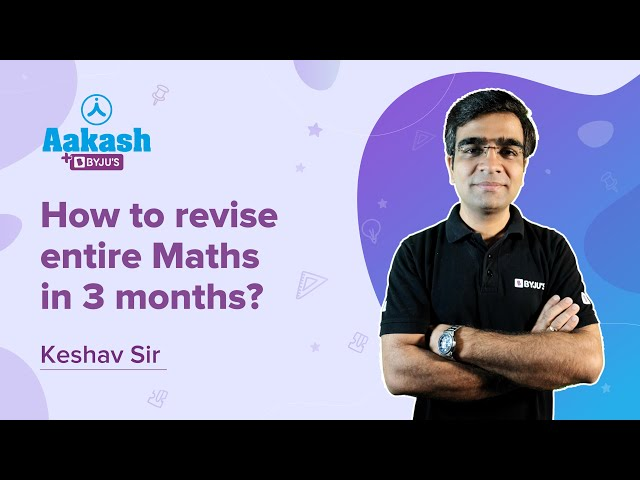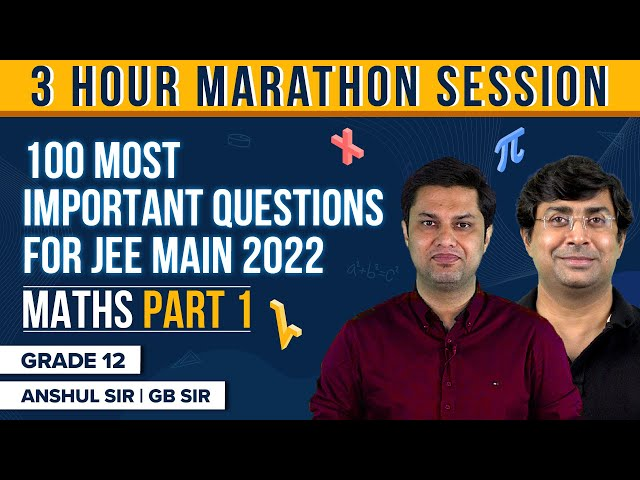# IIT JEE Mathematics Important Topics for JEE ExamsMathematics is the foundation of complex engineering calculations. In JEE exams, most of the questions asked in the Mathematics section are generally based on the formulas and their applications. You need to practice a lot for scoring well in this section. The more problems you solve, the better will be your speed and accuracy. It would be impossible to score by just memorizing and reading formulas in mathematics. Try to master all the key concepts by visualizing and apply them to the real word problems.

In JEE, there are a lot of chapters included in Mathematics syllabus and unlike Chemistry or Physics, there is nothing called less important or very important in mathematics as most of the chapters are interlinked. Therefore you can’t skip any particular section to focus more on any other section.

## JEE Mathematics Syllabus

The JEE syllabus for mathematics broadly consists of the following six topics:

1. Calculus: This unit is very crucial for both JEE Main and JEE Advanced mainly because of its vastness and level of difficulty. In every engineering Entrance Examination, you could expect several numbers of questions from this unit. You don’t have to worry much about JEE Mathematics if you develop a stronghold in this unit, therefore, you need to practice and prepare well for this unit. Important subtopics included in calculus are Limits, Differentiability and Continuity, Relationship between Continuity & Differentiability, Differentiation, Integration, Definite Integrals, Integration by Parts, properties of definite integrals, Rate Measurement, Tangents and Normal, Maxima and Minima, Differential Equations and Area under curves.

2. Co-ordinate Geometry: This unit is all about understanding some geometrical shapes like circles, parabola, hyperbola, and more. Questions from this unit are generally based on the application of formulas which makes it quite interesting and for most of the students it is a high-scoring unit. You can fetch maximum marks from this section. Focus more on Parabola, Circle, Ellipse, straight lines, and Hyperbola. Among all these, Straight Lines and Circles are the most important ones.

3. 3D Geometry and Vectors: This unit is quite complex and conceptual. Its weightage in JEE is somewhat similar to Coordinate Geometry. It also includes complex numbers along with some parts of geometry. You can easily score well from this section if you understand certain complex concepts of vectors and 3D geometry properly. Try to develop a stronghold on basics and focus more on understanding this unit through visualization.

4. Algebra: This unit is quite interesting and certain important topics like Logarithms, Matrices, and Determinants, and Quadratic Equations are quite easy to understand and are among the favourites for many students. Several times in JEE Main and Advanced there were few questions from Complex Number merged with the vectors. In JEE Advanced, you can expect a passage-type question from matrices and determinants and it might be the easiest one to answer. You can also expect a few conceptual questions from Permutations and Combinations. Formula-based questions are generally asked from Sequence and Series and Binomial Theorem. Therefore, by avoiding silly mistakes you could easily score good marks from this portion.

5. Trigonometry: It is one of the most important topics for JEE Main. Inverse Trigonometry, Trigonometric functions, and trigonometric ratios are some of the most imperative topics where you need to focus on. Since several numbers of questions are asked from this unit, therefore, getting the knack of some basic trigonometric concepts could surely benefit a JEE aspirant. For scoring well in this section you need to memorize all the trigonometric formulas along with their graphs and substitutions.

### Check IIT JEE Maths Important Topics

Now, let’s take a look at JEE Mathematics section 2019 that includes important topics for JEE Main (Paper 1) and JEE Advanced (Paper 1 and 2).

## Sure Shot Topics for Mathematics – JEE Main## Tips to Revise Maths JEE Main Syllabus in 3 Months### 100 Most Important Maths JEE Main Questions – Part 1### JEE Main 2019 Maths Section

The maths section in JEE Main 2019 exam had questions that were set on a moderate level. However, this section had problems that needed calculations and candidates found that it took a longer time than usual to solve all the questions. On breaking up the number of questions and the topics they were based on, algebra had 9 questions, coordinate geometry had 8 questions, calculus had 9 questions, and there were 4 questions from probability, mathematical reasoning, and statistics respectively. In terms of difficulty, 4 questions were difficult, 13 were on a moderate level and another 13 questions were easy.

Let us look at the topic-wise distribution of questions and weightage of marks below.

 Maths Topics No of Questions Weightage of Marks Matrices and Determinants 2 8 Vector Algebra 2 8 Three Dimensional Geometry 2 8 Complex numbers and Quadratic Equation 2 8 Limits, Continuity and Differentiability 3 12 Integral Calculus 3 12 Coordinate Geometry 5 20 Trigonometry 1 4 Statics and Dynamics 1 4 Statistics and Probability 2 8 Sets, Relation and Function 1 4 Sequences and Series 1 4 Binomial Theorem and Its Application 1 4 Differential Equation 1 4 Differential Calculus 1 4 Permutations and Combinations 1 4 Mathematical Reasoning 1 4 Total 30 120

The important chapters covered in the question paper are;

• Integration
• Complex Numbers
• Matrices and Determinants
• Circle
• 3D Geometry
• Binomial Theorem
• Application of Derivatives
• Probability and Statistics

### JEE Main 2018 Maths Section

The maths section in JEE Main 2018 question paper was deemed to be difficult as students and experts likewise, felt that the question required lengthy calculations and was time-consuming. There were a total of 20 questions set from the 11th standard syllabus while 10 questions were asked from the 12th standard syllabus. There were a total of 30 questions which included 3 difficult ones, 13 easy ones and 14 moderate questions.

 Important Topics Distribution Of Marks Algebra 56 Calculus 28 Conic 16 Vector Algebra 12 Trigonometry 8

### JEE Advanced 2018 Maths Section

The maths section in JEE Advanced 2018 question paper was on a moderate to difficult level. In paper 1 the mathematics section had questions that were concept-based and tricky. There were 16 questions set from the 12th standard syllabus. Paper 2 consisted of questions that were more formula-based and conceptual. 6 questions came from the 11th standard syllabus and 12 questions were asked from the 12th standard syllabus.

 Maths Topics No of Questions Weightage of Marks Fundamentals of Mathematics 1 2.50% Limit, Continuity & Differentiability 1 2.50% Application of Derivatives 1 3.33% Methods of Differentiation 1 3.33% Ellipse 1 3.33% Solution of Triangle 1 3.33% Inverse Trigonometric function 1 2.50% Trigonometric Ratio & identities 1 2.50% Sequence & Series 1 2.50% Algebra 1 2.50% Binomial Theorem 1 2.50% Hyperbola 1 2.50% Complex Numbers 2 6.67% Permutation & Combination 2 5.00% Functions 2 5.00% Vector 4 10.83% Vector and 3D 4 10.83% Differential equation 5 15.00% Coordinate Geometry 5 14.17% Differential Calculus 5 14.17% Integral Calculus 8 22.50%

To know more about JEE exams and get the latest notifications as well as study materials, preparation tips, and expert advice keep visiting BYJU’S.